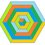# Okay, let's try this.

If you came here looking for new problems, then the answer is probably no. (Except if you've never solved these before, then don't read the notes after them.) But this note is an experimental test of this format (and me rambling about these problems without actually solving them).

(This was inspired by Varsha Dani. Thank you!)

Problem 2:

What is the minimum value of $q$ such that there are always three elements $x_m$, $x_n$, $x_p$ of the set $\mathbb A = \{x_1, x_2, \cdots, x_{q - 1}, x_q\} \in \{1; 2; \cdots; 1233; 1234\}$ such that $x_n^2 - 2x_px_m < x_p^2 + x_m^2 < x_n^2 + 2x_px_m$ (with every possible combination of the set $\mathbb A$)?

We have that

$\large x_n^2 - 2x_px_m < x_p^2 + x_m^2 < x_n^2 + 2x_px_m \implies |x_p - x_m| < x_n < x_p + x_m$

That means $x_m$, $x_n$, $x_p$ are three sides of a triangle. A similar property of a triangle is that if $a$ is the longest side of the triangle side $a$, $b$ and $c$ then $a < b + c$.

We can find out the minimum value of $q$ using induction with the Fibonacci sequence. Why the Fibonacci sequence, you ask? Because first of all, the Fibonacci numbers are the terms of a sequence of integers in which each term is the sum of the two previous terms with or $F_n = F_{n - 1} + F_{n - 2}$ ($n \in \mathbb N$ and $n \ge 2$). And secondly, the few Fibonacci numbers match the first few numbers from 1 to 1234.

More specifically, we'll the set $\{1; 2; 3; 5; 8; \cdots; 987\}$, which has 15 elements.

Now we have to prove that set $\mathbb A$ with a minimum of 16 elements must have three numbers $a$, $b$ and $c$ such that $a > b > c$ and $a < b + c$. Those 16 elements are $x_1 < x_2 < \cdots x_{15} < x_{16}$. It is true that

We have that

\large \left\{ \begin{aligned} x_4 &\ge x_3 + x_2 \ge 2x_2 + x_1\\ x_5 &\ge x_4 + x_3 \ge 3x_2 + 2x_1\\ \cdots\\ x_{15} &\ge x_{14} + x_{13} \ge 377x_2 + 233x_1\\ x_{16} &\ge x_{15} + x_{14} \ge 610x_2 + 377x_1\\ \end{aligned} \right.

Plugging in $x_1 \ge 1$ and $x_2 \ge 2$, $x_{16} \ge 610x_2 + 377x_1 \ge 1597 \notin A$, which is incorrect.

So the answer is 16. Check Mark Henning's more detailed explanation. It's better.

Problem 3:

There was a statement on a page on the book.

"$x_1$ and $x_2$ are the roots of the equation $\square_1 x^2 - mx + \square_2 = 0$, where $\square_1 \in \{m, n\}$ and $\square_2 \in \{m, n\}$ (with $mn > 0$ and $x_1 > x_2$). It is true that $\sqrt{\dfrac{x_1}{x_2}} + \triangle_1\sqrt{\dfrac{x_2}{x_1}} + \triangle_2\sqrt{\dfrac{m}{n}} = 0$, where $\triangle_1 \in \{-1, 1\}$ and $\triangle_2 \in \{-1, 1\}$."

Which of the following options is correct?

Option 1: $\square_1 = m, \square_2 = n, \triangle_1 = 1, \triangle_2 = -1$

Option 2: $\square_1 = m, \square_2 = n, \triangle_1 = -1, \triangle_2 = 1$

Option 3: $\square_1 = n, \square_2 = n, \triangle_1 = 1, \triangle_2 = -1$

Option 4: $\square_1 = n, \square_2 = n, \triangle_1 = 1, \triangle_2 = 1$

Option 5: $\square_1 = n, \square_2 = m, \triangle_1 = -1, \triangle_2 = 1$

Option 6: $\square_1 = n, \square_2 = m, \triangle_1 = 1, \triangle_2 = -1$

Option 7: $\square_1 = n, \square_2 = n, \triangle_1 = -1, \triangle_2 = 1$

Option 8: $\square_1 = n, \square_2 = n, \triangle_1 = -1, \triangle_2 = -1$

With elimination, you can work out can Option 6 is the answer. I can't really go into detail of why Option 6 is the answer. But what I can do is prove the statement is correct.

We have that $x_1 + x_2 = x_1x_2 = \dfrac{b}{a} = \dfrac{ab}{a^2} > 0$, which means $x_1$ and $x_2$ are positives, or $\sqrt{\dfrac{x_1}{x_2}}$, $\sqrt{\dfrac{x_2}{x_1}}$ and $\sqrt{\dfrac{b}{a}}$ are defined.

Also,

$\large \sqrt{\dfrac{x_1}{x_2}} + \sqrt{\dfrac{x_2}{x_1}} = \dfrac{x_1 + x_2}{\sqrt{x_1x_2}} = \dfrac{\dfrac{b}{a}}{\sqrt{\dfrac{b}{a}}} = \sqrt{\dfrac{b}{a}} \implies \sqrt{\dfrac{x_1}{x_2}} + \sqrt{\dfrac{x_2}{x_1}} - \sqrt{\dfrac{b}{a}} = 0$

And that's all I have to say! Thanks again to Varsha Dani. Here's her discussion: "AVL Trees meet Fibonacci Numbers".Note by Thành Đạt Lê
1 year, 9 months ago

This discussion board is a place to discuss our Daily Challenges and the math and science related to those challenges. Explanations are more than just a solution — they should explain the steps and thinking strategies that you used to obtain the solution. Comments should further the discussion of math and science.

When posting on Brilliant:

• Use the emojis to react to an explanation, whether you're congratulating a job well done , or just really confused .
• Ask specific questions about the challenge or the steps in somebody's explanation. Well-posed questions can add a lot to the discussion, but posting "I don't understand!" doesn't help anyone.
• Try to contribute something new to the discussion, whether it is an extension, generalization or other idea related to the challenge.

MarkdownAppears as
*italics* or _italics_ italics
**bold** or __bold__ bold
- bulleted- list
• bulleted
• list
1. numbered2. list
1. numbered
2. list
Note: you must add a full line of space before and after lists for them to show up correctly
paragraph 1paragraph 2

paragraph 1

paragraph 2

[example link](https://brilliant.org)example link
> This is a quote
This is a quote
    # I indented these lines
# 4 spaces, and now they show
# up as a code block.

print "hello world"
# I indented these lines
# 4 spaces, and now they show
# up as a code block.

print "hello world"
MathAppears as
Remember to wrap math in $$ ... $$ or $ ... $ to ensure proper formatting.
2 \times 3 $2 \times 3$
2^{34} $2^{34}$
a_{i-1} $a_{i-1}$
\frac{2}{3} $\frac{2}{3}$
\sqrt{2} $\sqrt{2}$
\sum_{i=1}^3 $\sum_{i=1}^3$
\sin \theta $\sin \theta$
\boxed{123} $\boxed{123}$